## Background: Mazezam's complexity

Mazezam is a puzzle game written by Malcolm Tyrrell, and back in 2008 I constructed what I thought was an argument for why this game was 'NP-complete'. This means two things:

• It is in NP.
• That it is 'NP-hard', meaning that if Mazezam can be solved in polynomial time, then so can any other problem in NP.

I recently received an email from Aaron Williams pointing out that what I had actually done was show the second part only. I had not shown that Mazezam was in NP.

I had initially thought this part was obvious — if somebody proposes a solution to a level, just try simulating the given sequence of moves and check the hero does indeed make it to the exit. However, Aaron pointed out I had missed an important detail of the 'polynomial time verification' formulation of NP. The definition of NP, in this formulation, is given in lectures notes from CMU's Algorithms course:

A problem is in NP if there is a polynomial-time algorithm V(I,X) such that:

• If I is a YES-instance, then there exists X such that V(I,X) = YES.
• If I is a NO-instance, then for all X, V(I,X) = NO.

(Here, X is a 'witness' to the solubility of I, for example a description of the solution to I.) Furthermore, X should have length polynomial in size of I (since we are really only giving V time polynomial in the size of the instance, not the combined size of the instance and solution).

It was the 'X should have length polynomial in size of I' part that I had overlooked. Aaron conjectured that

the shortest series of button presses (i.e., down, right, right, up, etc.) to solve a given level could, in theory, be exponential in length with respect to the size of the puzzle.

## An exponential level family

I thought it would be interesting to try to construct a family of levels with this property. I thought that something like the Towers of Hanoi or the Chinese Rings ought to work. The solution to the Chinese Rings led me to the idea of forcing the player to work their way through all possible states of a Gray code counter.

The building blocks are similar to those in my original write-up. There will be one movable row per bit in the modelled counter. The player can change each such row between a position representing '`0`' and a position representing '`1`'. However, the only changes possible will be those matching the permissible changes to a Gray code counter:

• The least significant bit, 'bit 0', can be changed at any time.
• Any other bit can only be changed if the next-less-significant bit is a '`1`' and all lower-significance bits (if any) are '`0`'.

Except when the counter is in its starting (`00...00`) or ending (`10..00`) state, there is always a bit position satisfying the second bullet.

For example, if an 8-bit counter is currently `01011000`, then the permissible changes are:

• `01011000``01011001` (change least significant bit);
• `01011000``01001000` (change bit 4, because bit 3 is `1` and bits 2, 1, and 0 are `0`).

I achieve these constraints in a Mazezam level by using the same vertical corridors as previously to enforce the requirements, joined to a modified corridor to allow the toggling.

The idea is best illustrated with an example.

## Demo

We now illustrate the scheme for a 6-bit counter.

The level below forces the player to go through the complete 6-bit Gray code counter from its initial state of `000000` to its final state of `100000`, at which point the player can escape out of the bottom-right. The player moves in the black areas. The light-grey rows are movable and the dark-grey rows are fixed. The bottom light row, (call it `b0`), is 'bit 0', the least-significant bit, and so on up to the top light row (`b5`), which is 'bit 5', the most-significant bit.

A light row in its leftward position means that bit is '`0`'; in its rightwards position means '`1`'. All rows therefore start off in their leftward position.

The bulk of the level is made of horizontally-stacked reflected 'J's. The long (left, 'open' at top) arm of a reflected J allows passage through it under certain conditions, but does not allow changing any rows while still being able to get to the bottom. The short (right, dead-end) arm lets you toggle one particular bit, while forcing you to leave all other bits alone. If you do toggle that bit, you can still get out the long (left) arm.

The 'J's are configured to embody the Gray code requirements:

The leftmost reflected 'J' allows you to flip `b0` at any time. The second from the left allows you to flip `b1` whenever `b0`=`1`. The third from left allows you to flip `b2` whenever `b1`=`1` and `b0`=`0`. The fourth from left lets you flip `b3` if `b2`=`1`, `b1`=`0`, and `b0`=`0`. And so on. The rightmost corridor allows you to successfully exit the level if the bit-vector is `100000`.

Use the `Start` button to animate the solution to the level; `Reset` to reset the level. The radio buttons control the animation's speed.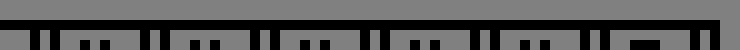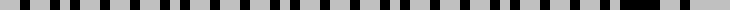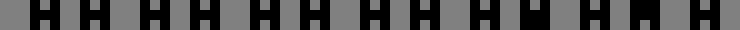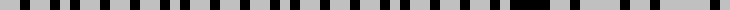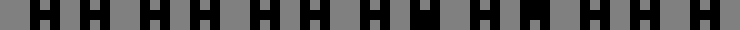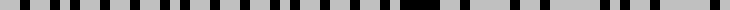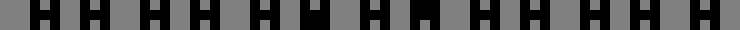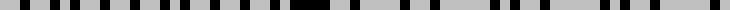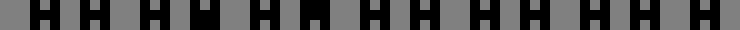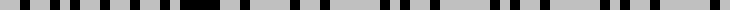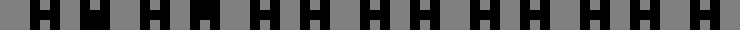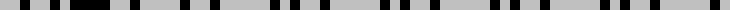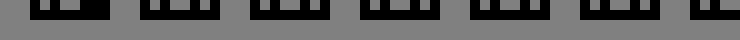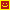Counter value: 0 0 0 0 0 0 Number of moves: 0
Slow Fast Warp

(Thanks to Pedro Gimeno for reporting a bug where the demo did not behave correctly in some browsers.)

## Conclusion

The scheme could be expanded to an arbitrary number of bits, with the result being a level whose width and height are both linear in the number of bits in the counter, but whose solution is exponential in the number of bits.

## Source code

Available on github: bennorth/exponential-mazezam.